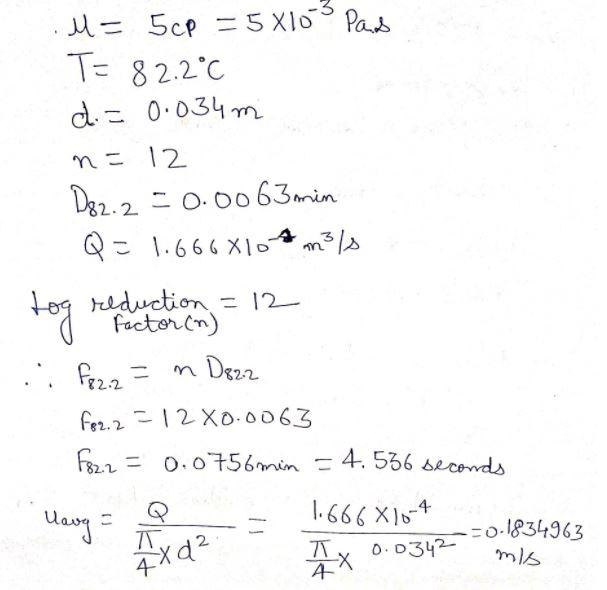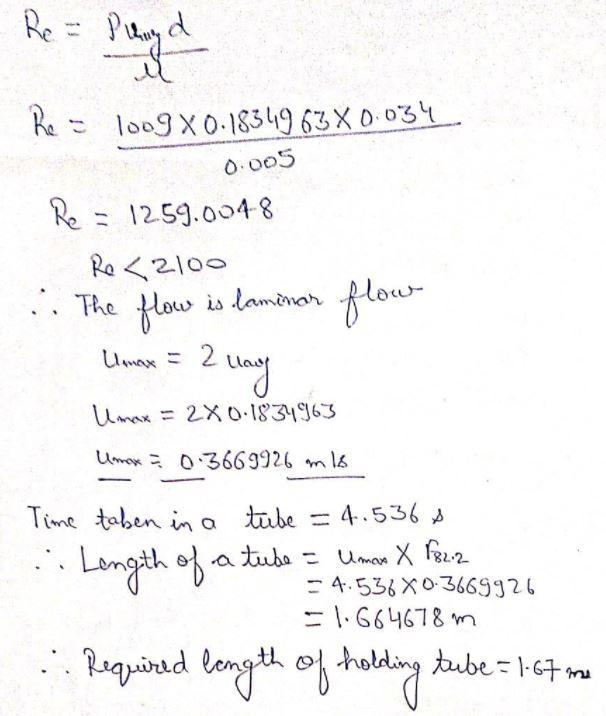# A fluid food has a viscosity of 5 cp and density of 1009 kg/m³. It is pasteurised in a continuous system that involves heating at 82.2°C, holding in a pipe (diameter = 0.034 m; crossectional area = 9.079×10+ m²) and cooling. For this heating process a SV of 12 for Staphylococcus aureus is desired. The D822 value is equal to 0.0063 min. Calculate the length (in m) of holding tube for a flow rate of 1.666×10+ m³/s.

Question-AnswerCategory: Chemical EngineeringA fluid food has a viscosity of 5 cp and density of 1009 kg/m³. It is pasteurised in a continuous system that involves heating at 82.2°C, holding in a pipe (diameter = 0.034 m; crossectional area = 9.079×10+ m²) and cooling. For this heating process a SV of 12 for Staphylococcus aureus is desired. The D822 value is equal to 0.0063 min. Calculate the length (in m) of holding tube for a flow rate of 1.666×10+ m³/s.

A fluid food has a viscosity of 5 cp and density of 1009 kg/m³. It is pasteurised in a continuous system that involves heating at 82.2°C, holding in a pipe (diameter = 0.034 m; crossectional area = 9.079×10+ m²) and cooling. For this heating process a SV of 12 for Staphylococcus aureus is desired. The D822 value is equal to 0.0063 min. Calculate the length (in m) of holding tube for a flow rate of 1.666×10+ m³/s.